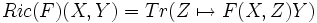# Ricci map

(diff) ← Older revision | Latest revision (diff) | Newer revision → (diff)

alt text="BEWARE"This term is nonstandard and is being used locally within the wiki. For its use outside the wiki, please define the term when using it.

## Definition

The Ricci map is a map from the space of$(1,3)$-tensors on a manifold to the space of$(0,2)$-tensors, defined as follows:$Ric(F)(X,Y) = Tr(Z \mapsto F(X,Z)Y)$

For instance, the Ricci map applied to the Riemann curvature tensor, gives the Ricci curvature tensor.

## Facts

### Riemannian curvature space

When looking at the Ricci map, we often consider its restriction on the Riemannian curvature space at each point, rather than on the space of all$(1,3)$-tensors. The kernel of this map is termed the Weyl curvature space and the image of this map is termed the Ricci curvature space.